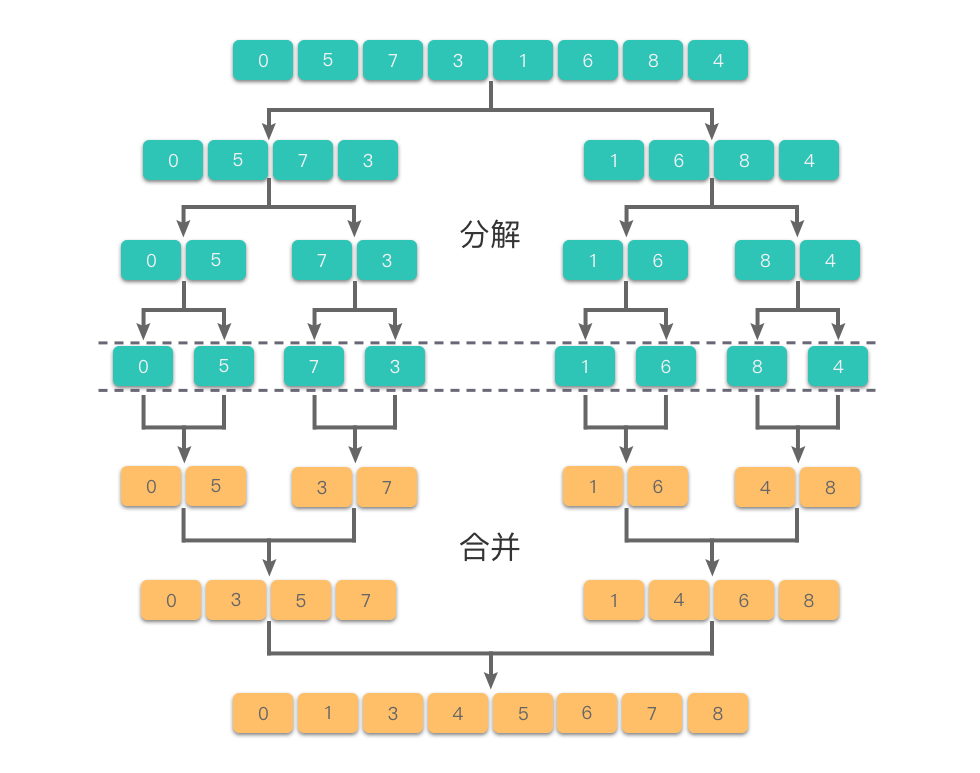# 剑指 Offer 51. 数组中的逆序对#

• 标签：树状数组、线段树、数组、二分查找、分治、有序集合、归并排序
• 难度：困难

## 题目大意 #

• 逆序对：在数组中的两个数字，如果前面一个数字大于后面的数字，则这两个数字组成一个逆序对。
• $0 \le nums.length \le 50000$。

• 示例 1：
 1 2  输入: [7,5,6,4] 输出: 5 

## 解题思路 #

### 思路 1：归并排序 #1. 使用全局变量 cnt 来存储逆序对的个数。然后进行归并排序。
2. 分割过程：先递归地将当前序列平均分成两半，直到子序列长度为 1
1. 找到序列中心位置 mid，从中心位置将序列分成左右两个子序列 left_arrright_arr
2. 对左右两个子序列 left_arrright_arr 分别进行递归分割。
3. 最终将数组分割为 n 个长度均为 1 的有序子序列。
3. 归并过程：从长度为 1 的有序子序列开始，依次进行两两归并，直到合并成一个长度为 n 的有序序列。
1. 使用数组变量 arr 存放归并后的有序数组。
2. 使用两个指针 left_iright_i 分别指向两个有序子序列 left_arrright_arr 的开始位置。
3. 比较两个指针指向的元素：
1. 如果 left_arr[left_i] <= right_arr[right_i]，则将 left_arr[left_i] 存入到结果数组 arr 中，并将指针移动到下一位置。
2. 如果 left_arr[left_i] > right_arr[right_i]，则 记录当前左子序列中元素与当前右子序列元素所形成的逆序对的个数，并累加到 cnt 中，即 self.cnt += len(left_arr) - left_i，然后将 right_arr[right_i] 存入到结果数组 arr 中，并将指针移动到下一位置。
4. 重复步骤 3，直到某一指针到达子序列末尾。
5. 将另一个子序列中的剩余元素存入到结果数组 arr 中。
6. 返回归并后的有序数组 arr
4. 返回数组中的逆序对的总数，即 self.cnt

### 思路 1：代码 #

  1 2 3 4 5 6 7 8 9 10 11 12 13 14 15 16 17 18 19 20 21 22 23 24 25 26 27 28 29 30 31 32 33 34 35 36 37 38 39 40  class Solution: cnt = 0 def merge(self, left_arr, right_arr): # 归并过程 arr = [] left_i, right_i = 0, 0 while left_i < len(left_arr) and right_i < len(right_arr): # 将两个有序子序列中较小元素依次插入到结果数组中 if left_arr[left_i] <= right_arr[right_i]: arr.append(left_arr[left_i]) left_i += 1 else: self.cnt += len(left_arr) - left_i arr.append(right_arr[right_i]) right_i += 1 while left_i < len(left_arr): # 如果左子序列有剩余元素，则将其插入到结果数组中 arr.append(left_arr[left_i]) left_i += 1 while right_i < len(right_arr): # 如果右子序列有剩余元素，则将其插入到结果数组中 arr.append(right_arr[right_i]) right_i += 1 return arr # 返回排好序的结果数组 def mergeSort(self, arr): # 分割过程 if len(arr) <= 1: # 数组元素个数小于等于 1 时，直接返回原数组 return arr mid = len(arr) // 2 # 将数组从中间位置分为左右两个数组。 left_arr = self.mergeSort(arr[0: mid]) # 递归将左子序列进行分割和排序 right_arr = self.mergeSort(arr[mid:]) # 递归将右子序列进行分割和排序 return self.merge(left_arr, right_arr) # 把当前序列组中有序子序列逐层向上，进行两两合并。 def reversePairs(self, nums: List[int]) -> int: self.cnt = 0 self.mergeSort(nums) return self.cnt 

### 思路 1：复杂度分析 #

• 时间复杂度：$O(n \times \log_2n)$。
• 空间复杂度：$O(n)$。

### 思路 2：树状数组 #

1. 按序列从左到右将值为 nums[i] 的元素当作下标为nums[i]，赋值为 1 插入树状数组里，这时，比 nums[i] 大的数个数就是 i + 1 - query(a)
2. 将全部结果累加起来就是逆序数了。

### 思路 2：代码 #

  1 2 3 4 5 6 7 8 9 10 11 12 13 14 15 16 17 18 19 20 21 22 23 24 25 26 27 28 29 30 31 32 33 34 35 36  import bisect class BinaryIndexTree: def __init__(self, n): self.size = n self.tree = [0 for _ in range(n + 1)] def lowbit(self, index): return index & (-index) def update(self, index, delta): while index <= self.size: self.tree[index] += delta index += self.lowbit(index) def query(self, index): res = 0 while index > 0: res += self.tree[index] index -= self.lowbit(index) return res class Solution: def reversePairs(self, nums: List[int]) -> int: size = len(nums) sort_nums = sorted(nums) for i in range(size): nums[i] = bisect.bisect_left(sort_nums, nums[i]) + 1 bit = BinaryIndexTree(size) ans = 0 for i in range(size): bit.update(nums[i], 1) ans += (i + 1 - bit.query(nums[i])) return ans 

### 思路 2：复杂度分析 #

• 时间复杂度：$O(n \times \log_2n)$。
• 空间复杂度：$O(n)$。